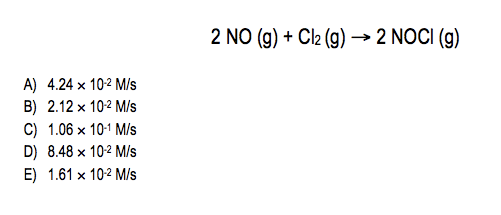# Problem: Given the following balanced equation, if the rate of Cl2 loss is 4.24 × 10-2 M/s, what is the rate of formation of NOCl?

🤓 Based on our data, we think this question is relevant for Professor Toto's class at San Diego Mesa College.

###### Problem Details

Given the following balanced equation, if the rate of Cl2 loss is 4.24 × 10-2 M/s, what is the rate of formation of NOCl?What scientific concept do you need to know in order to solve this problem?

Our tutors have indicated that to solve this problem you will need to apply the Average Rate of Reaction concept. You can view video lessons to learn Average Rate of Reaction. Or if you need more Average Rate of Reaction practice, you can also practice Average Rate of Reaction practice problems.

What is the difficulty of this problem?

Our tutors rated the difficulty ofGiven the following balanced equation, if the rate of Cl2 lo...as low difficulty.

How long does this problem take to solve?

Our expert Chemistry tutor, Jules took 2 minutes and 9 seconds to solve this problem. You can follow their steps in the video explanation above.

What professor is this problem relevant for?

Based on our data, we think this problem is relevant for Professor Toto's class at San Diego Mesa College.